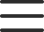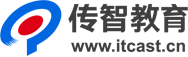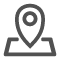# C++培训之四种程序结构

c = a；
a = b；
b = c；

a = b；
c = a；
b =c；

## （2） 分支结构

### ②if(条件){分支1} else {分支2}

1. main()
2. {
3. int a,b,c,d,x,y;
4. printf("Please put the number of a,b&c from the quadratic equation of one variable one by one\n");
5. scanf("%d%d%d",&a,&b,&c);
6. d=b*b-4*a*c;
7. if(d<0)
8. {
9. printf("NO Root!Wrong!\n");
10. }
11. else
12. {
13. y=-b-sqrt(d)/2*a;
14. x=-b+sqrt(d)/2*a;
15. printf("The 1st equation root=%d\nThe 2nd equation root=%d",y,x);
16. }

### ③IF嵌套分支语句：

1. if(条件1) {分支1}
2. else if（条件2） {分支2}
3. else if（条件3） {分支3}
4. ……
5. else if（条件n） {分支n}
6. else {分支n+1}
7. FOR嵌套，其语句格式为：
8. for(初值A；范围A；步长A)
9. {
10. for(初值B；范围B；步长B）
11. {
12. 循环体
13. }
FOR嵌套例子：九九乘法表
1. main()
2. {
3. int a,b,c;
4. for(a=1;a<=9;a++)
5. {
6. for(b=1;b<=a;b++)
7. {
8. c=b*a;
9. printf("%dx%d=%d ",b,a,c);
10. }
11. printf("\n");
12. }

## (4)模块化程序结构

C语言的模块化程序结构用函数来实现，即将复杂的C程序分为若干模块，每个模块都编写成一个C函数，然后通过主函数调用函数及函数调用函数来实现一大型问题的C程序编写，因此常说：C程序=主函数+子函数。 因此，对函数的定义、调用、值的返回等中要尤其注重理解和应用，并通过上机调试加以巩固。

本文版权归传智播客C++培训学院所有，欢迎转载，转载请注明作者出处。谢谢！